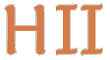# Hilbert II

 English Deutsch
 introduction news mathematics QEDEQ planning download glossary development prototype links contact site map
IntroductionThe goal of Hilbert II is decentralised access to verified and readable mathematical knowledge. As its name already suggests, this project is in the tradition of Hilbert's program.

Hilbert II wants to become a free, world wide mathematical knowledge base that contains mathematical theorems and proofs in a formal correct form. All belonging documents are published under the GNU Free Documentation License. We aim to adapt the common mathematical argumentation to a formal syntax. That means, whenever in mathematics a certain kind of argumentation is often used we will look forward to integrate it into the formal language of Hilbert II. This formal language is called the QEDEQ format.

Hilbert II provides a program suite that enables a mathematician to put theorems and proofs into that knowledge base. These proofs are automatically verified by a proof checker. Also texts in "common mathematical language" can be integrated. The mathematical axioms, definitions and propositions are combined to so called QEDEQ modules. Such a module could be seen as a mathematical textbook which includes formal correct proofs. Because this system is not centrally administrated and references to any location in the internet are possible, a world wide mathematical knowledge base could be build. Any proof of a theorem in this "mathematical web" could be drilled down to the very elementary rules and axioms. Think of an incredible number of mathematical textbooks with hyperlinks and each of its proofs could be verified by Hilbert II. For each theorem the dependency of other theorems, definitions and axioms could be easily derived.

The main project is still in development but you can already download the current application. It has a GUI and can load QEDEQ module files located anywhere in the internet. It can transform QEDEQ modules into LaTeX and UTF-8 text files. Most PDF documents of this web site were generated in fact by this application. It even can check simple formal proofs. For set theory there are simple discrete models integrated so the application can check if a formula is valid. In new QEDEQ modules you can use QEDEQ modules that exist already in the web just by referencing them.

For further information see under state and planning and development.

There also exists a working prototype called Principia Mathematica II. It is fully capable of first order predicate logic and shows the main features and functionality of Hilbert II.

update 2014-01-20 09:20:48+0100